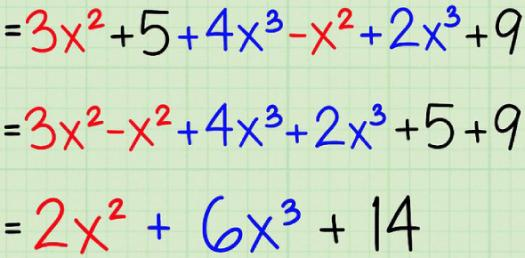# Year 8 Maths 3.1 Algebra - Variables And Expressions

10 Questions | Total Attempts: 1177SettingsFor those of you who love all things mathematics especially graphs and algebra that measures the strength of materials then this is the quiz for you. If you are preparing for exams, and want to test your knowledge, try it out.

• 1.
What is the algebraic expression to represent m divided by 3
• A.
• B.
• C.

3m

• D.

M3

• 2.
What is the algebraic expression to represent two lots of p
• A.
• B.
• C.
• D.
• 3.
What is the algebraic expression to represent y less than twenty
• A.

Y + 20

• B.

Y - 20

• C.

20 + y

• D.

20 - y

• 4.
A ___________________ describes an unknown amount in an algebraic expression or equation. Can be represented by letters or symbols (e.g. a, y, ) and can be called pronumerals (which means 'in place of' a number).
• A.

Coefficient

• B.

Constant

• C.

Equation

• D.

Expression

• E.

Term

• F.

Variable

• 5.
The following are examples of  ___________________ .
• A.

Coefficients

• B.

Constants

• C.

An equation

• D.

An expression

• E.

Terms

• F.

Variables

• 6.
8x means that x is multiplied 8 times.  The number in front of the term x is called the _______________.
• A.

Coefficient

• B.

Constant

• C.

An equation

• D.

An expression

• E.

Term

• F.

Variable

• 7.
A number that is written by itself is called a _______________. E.g. in the expression 3x + 6, the 6 is a _____________.
• A.

Coefficient

• B.

Constant

• C.

An equation

• D.

An expression

• E.

Terms

• F.

Variable

• 8.
Two expressions joined together by an equals sign is E.g.  3x + 6 = 2x - 4.
• A.

Coefficients

• B.

Constants

• C.

An equation

• D.

An expression

• E.

Terms

• F.

Variables

• 9.
When two or more terms are added or subtracted together, it creates ___________________. E.g. 3x + 4y
• A.

Coefficients

• B.

Constants

• C.

An equation

• D.

An expression

• E.

Terms

• F.

Variables

• 10.
What is the algebraic expression to represent ten more than x?
• A.

10x

• B.

X - 10

• C.

X + 10

Related TopicsBack to top